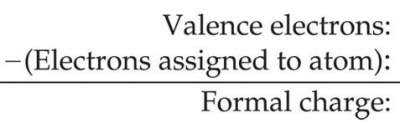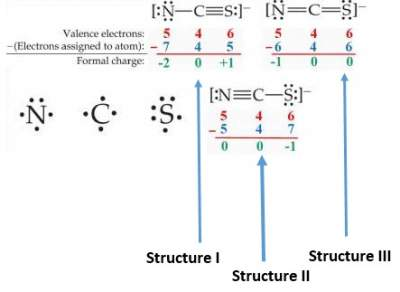# Drawing Lewis Structures

## Introduction

These are structures built using Lewis Symbols of its elements based on certain rules:
• Add the valence electrons of all atoms in the compound.
• Write symbols for the atoms and show which atoms are connected to which, and connect them with a single bond.
• Complete the octet for atoms bonded to the central atom.
• Place leftover electrons on the central atom.
• If there are not enough electrons to give the central atom an octet, try multiple bonds.

### Exercises on Drawing Lewis Structures and Adding the Valence Electrons

Exercise I on Drawing Lewis Structures and Adding the Valence Electrons

Check your answers here: Solution to the Exercise I on Drawing Lewis Structures and Adding the Valence Electrons

Exercise II on Drawing Lewis Structures and Adding the Valence Electrons

Check your answers here: Solution to the Exercise II on Drawing Lewis Structures and Adding the Valence Electrons

Exercise III on Drawing Lewis Structures and Adding the Valence Electrons

Check your answers here: Solution to the Exercise III on Drawing Lewis Structures and Adding the Valence Electrons

Exercise IV on Drawing Lewis Structures

Check your answers here: Solution to the Exercise IV on Drawing Lewis Structures

Exercise V on Drawing Lewis Structures

Check your answers here: Solution to the Exercise V on Drawing Lewis Structures

### Formula Charge

It is used to decide on the most stable Lewis Structure. It is calculated as follows:For every atom in the Lewis Structure, so that the summation of all formal charges gives the overall charge on the anion.
Hence, to identify the most stable structure from three structures shown in exercise IV, we apply the formula as illustrated below:Structure I is NOT possible since S has a POSITIVE formal charge, Which is not acceptable for a high electronegative element.
Structure II is NOT possible since S has a MORE NEGATIVE formal Charge than N, which is not acceptable since N > S in electronegativity.
Structure III is the most stable one.

### Exercises on Lewis Structures and Formula Charge

Exercise on Lewis Structures and Formula Charge

Check your answers here: Solution to the Exercise on Lewis Structures and Formula Charge